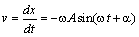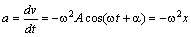# Physics Assignment Help With Energy in Kinematics of Simple Harmonic Motion

## 7.2 Kinematics of Simple Harmonic Motion

A particle has simple harmonic motion along an axis OX when its displacement x relative to the origin of the coordinate system is given as a function of time by the relation

x = A cos (wt + a) …(i)

The quantity (wt + a) is called the phase angle or simply the phase of the SHM, and a is the initial phase, i.e. the phase at t = 0. Although we have defined simple harmonic motion in terms of a cosine function, it may just as well as expressed in terms of a sine function. The only difference between the two forms is an initial phase difference of p/2. Since the cosine (or sine) function varies between a value of –1 and +1, the displacement of the particle varies between x = – A and x = + A. The maximum displacement from the origin, A, is the amplitude of the simple harmonic motion. The cosine function repeats itself every time the angle wt increases by 2p. Thus the displacement of the particle repeats itself after a time interval of 2p/w. Therefore, simple harmonic motion is periodic, and its period is

P = 2p/w

The frequency v of a simple harmonic motion is equal to the number of complete oscillations per unit time;

v = 1/P

frequency is measured in hertz. The quantity w, called the angular frequency of the oscillating particle, is related to the frequency by a relation similar to circular motion, namely

w = 2p/P = 2pv …(ii)

The velocity of the particle is…(iii)

which varies periodically between the values wA and –wA. Similarly, the acceleration, is given by…(iv)

and therefore varies periodically between the values + w2A and –w2A. This expression also indicates that : in simple harmonic motion the acceleration is proportional and opposite to the displacement.

### Email Based Assignment Help in Kinematics of Simple Harmonic Motion

We are the leading online Assignment Help provider. Find answers to all of your doubts regarding the Kinematics of Simple Harmonic Motion. Assignmenthelp.net provide homework, Assignment Help to the school, college or university level students. Our expert online tutors are available to help you in Kinematics of Simple Harmonic Motion. Our service is focused on: time delivery, superior quality, creativity and originality.